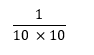• ## Accessibility

Open the accessibility toolbar to change fonts and contrast, access a dictionary, use a ruler and more

If you confident with positive integers, we are now going to learn to evaluate numbers using negative indices.

If you are not sure what these are, why not review these before you move forwards?

Think about the patterns you can see in the following table:

 Start with 1000 1000 10 x 10 x 10 103 Then divide by 10 100 10 x 10 102 Divide by 10 again 10 10 101 Divide by 10 again 1 1 100  Remember that any term to the power of 0 is 1 Divide by 10 again10-1 Divide by 10 again10-2 Divide by 10 again10-3

We can see that the pattern in these powers of 10 shows us that:Notice that we are, in fact, finding the 'reciprocal' of:e.g. Evaluate 2-3.

This means work out the value of:We have found the reciprocal of 23.

e.g. Evaluate 3-4.

This means work out the value of:We have found the reciprocal of 34.

In this activity, we will evaluate and calculate negative indices with negative numbers and fractions as we have seen in the examples above.

You may want to have a pen and paper handy before you start so that you can compare your working to our answers written by a maths teacher.

10 questions